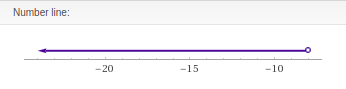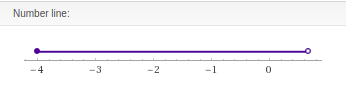# Solving Compound Inequalities

Compound inequalities are two inequalities that are joined by the word “and” or the word “or”. Solving a compound inequality means finding all values that make the compound inequality true.For compound inequalities join ed by the word “and” we look for solutions that are true for both inequalities. Fo compound inequalities joined by the word “or” we look for solutions that work for either inequality.

It is also possible to graph compound inequalities on a number line as well as indicate the final answer using interval notation. Below is a compound inequality with the line graph solutionSolving the answer is the same as a regular equation. Below is the number line for this answer.The empty circle at -8 means that -8 is not part of the solution. This means all values less than -8 are acceptable answers. This is why the line moves from right to left. All values less than -8 until infinity are acceptable answers. Below is the interval notation.The parentheses mean that the value next to it is not included as a solution. This corresponds to the empty circle over the -8 in the lin graph. If the value should be included such as with a less/greater than sign you would use a bracket.

Double Inequality

A double inequality is a more concise version of a compound inequality. The goal is to isolate the variable in the middle. Below is an exampleThis is not complex. We simply isolate x in the middle using appropriate steps. The number line and interval notation or as follows# [-4, 2/3)

This time there is a bracket next to -4 which means that -4 is also a potential solution. In addition, notice how the -4 has a filled circle on the number line. This is another indication that -4 is a solution.

Practical Application

You have signed up for internet access through your cell phone. Your bill is a flat \$49.00 per month please \$0.05 per minute for internet use. How many minutes can you use internet per month if you want to keep your bill somewhere between \$54-\$74 per month?

Below is the solution using a double inequalityThe answer indicates that you can spend anywhere from 100 to 500 minutes on the internet through your phone per month to stay within the budget. You can make the number line and develop the interval notation yourself.

Conclusion

Compound inequalities are useful for not only as an intellectual exercise. They can also be used to determine practical solutions that include more than one specific answer.